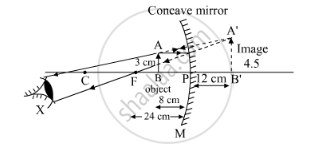Share

# An Object 3 Cm High is Placed at a Distance of 8 Cm from a Concave Mirror Which Produces a Virtual Image 4.5 Cm High: (I) What is the Focal Length of the Mirror? (Ii) What is the Position of Image? (Iii) Draw a Ray-diagram to Show the Formation of Image. - CBSE Class 10 - Science

ConceptRepresentation of Images Formed by Spherical Mirrors Using Ray Diagrams - Concave Mirror

#### Question

An object 3 cm high is placed at a distance of 8 cm from a concave mirror which produces a virtual image 4.5 cm high:

(i) What is the focal length of the mirror?
(ii) What is the position of image?
(iii) Draw a ray-diagram to show the formation of image.

#### Solution

Distance of the object from the mirror 'u' = -8 cm

Height of the object 'ho' = 3 cm
Height of the image 'hi' = 4.5 cm
We have to find the focal length of the mirror 'f' and distance of the image 'v'.
Using the magnification formula, we get

m=h_i/h-o=-v/u

m4.5/3=(-v)/(-8)

v=4.5xx8/3=12cm

Therefore, the distance of the image 'v' is 12 cm behind the mirror.

Now, using the mirror formula, we get
1/f=1/v+1/u=1/12+1/-8
1/f=2/24-3/24=-1/24
f =-24 cm

Thus, the focal length of the concave mirror 'f' is 24 cm.Is there an error in this question or solution?

#### APPEARS IN

Solution An Object 3 Cm High is Placed at a Distance of 8 Cm from a Concave Mirror Which Produces a Virtual Image 4.5 Cm High: (I) What is the Focal Length of the Mirror? (Ii) What is the Position of Image? (Iii) Draw a Ray-diagram to Show the Formation of Image. Concept: Representation of Images Formed by Spherical Mirrors Using Ray Diagrams - Concave Mirror.
S